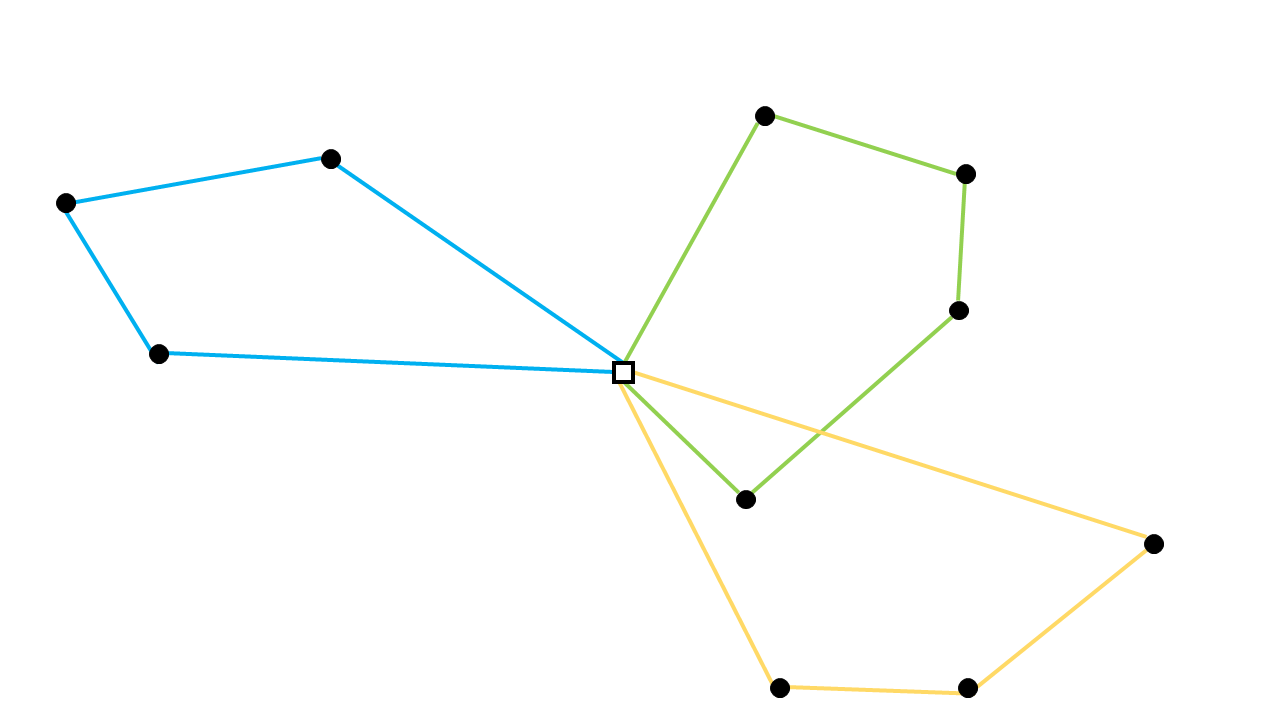# Capacitated Vehicle Routing Problem Library

There is a library in AIMMS that solves a Capacitated Vehicle Routing Problem (CVRP). This article explains how to use that library in your own model. There are different ways to formulate a CVRP. In the CVRP library there are four options to choose from, which will be mentioned in this article.

`AIMMS library download`

## Capacitated Vehicle Routing Problem

A CVRP deals with the following problem: A set is given with a depot and multiple costumers. The distances between their locations are known. A number of vehicles is available to serve the costumers. All costumers have a certain demand and the vehicles have the same maximum capacity. The shortest route for the vehicles must be found where all costumers get their demand. The vehicles all start and end at the depot.## How to use the Library

2. One of the required input arguments is `s_Formulations`. You should make this set a subset of `cvrpl::PossibleFormulations`. Which is a set inside the library with all possible formulations.

3. When you choose a formulation without time windows, the library can be called with the procedure `cvrpl::pr_NoTimeWindows`. If you choose the formulation with time windows, the library can be called with the procedure `cvrpl::pr_CVRPLibrary`. They have the following input and output arguments:

```cvrpl::pr_CapacitatedVehicleRoutingProblem
(s_Formulations, s_Nodes, p_NumberOfVehicles, p01_MaxorExact,
p_Distance, p_Demand, p_Capacity, p_TotalDistance, p01_x, p_BoundTotalDist,
sp_SolverStatus, sp_ProgramStatus, p_SolverTime);
```
```cvrpl::pr_CapacitatedVehicleRoutingProblemTimeWindows(
s_Formulations, s_Nodes, p_NumberOfVehicles, p01_MaxorExact,
p_Distance, p_Demand, p_Capacity, p_TWLowerBound, p_TWUpperBound, p_ServiceTime,
p_TotalDistance, p01_x, p_StartServing, p_BoundTotalDist, sp_SolverStatus,
sp_ProgramStatus, p_SolverTime);
```

## Input and output arguments

Input Arguments

Type

Index

Index Domain

s_Formulations

Set

s_Nodes

Set

i, j

p_NumberOfVehicles

Parameter

p01_MaxorExact

Parameter (binary)

p_Distance

Parameter

(i, j)

p_Demand

Parameter

( i )

p_Capacity

Parameter

( k )

p_TWLowerBound *

Parameter

( i )

p_TWUpperBound *

Parameter

( i )

p_ServiceTime *

Parameter

(i, j)

`s_Formulations` should contain the formulation you want to use to solve the problem, choosing from: ‘Explicit Dantzig-Fulkerson-Johnson’, ‘Miller-Tucker-Zemlin’, ‘Implicit Dantzig-Fulkerson-Johnson’ or ‘Time Windows’ The set `s_Nodes` contains the depot and all costumers. `p_MaxorExact` is a binary parameter that indicates whether `p_NumberOfVehicles` is a maximum or an exact amount. If `p_MaxorExact` is 0, then a maximum of `p_NumberOfVehicles` can be used. If `p_MaxorExact` is 1, then exactly `p_NumberOfVehicles` should be used. `p_Distance` describes the distance between two nodes. When there is no road between two nodes, you can just leave the value for that distance empty.

• These input arguments are only necessary when you use time windows. `p_TWLowerBound` and `p_TWUpperBound` indicate the time in between which a vehicle should arrive at node `i`. `p_ServiceTime` denotes the time it takes to get from node `i` to node `j`. It may include the service time at node `i`.

Output Arguments

Type

Index

Index Domain

p_TotalDistance

Parameter

p01_x

Parameter (binary)

(i, j, k) | i <> j

p_StartServing *

Parameter

( i )

p_BoundTotalDist

Parameter

sp_SolverStatus

String Parameter

sp_ProgramStatus

String Parameter

p_SolverTime

Parameter

`p_TotalDistance` is the total distance of the shortest route. `p01_x` is a binary variable with a value of `1` if the road from `i` to `j` is in the shortest route and is driven by vehicle `k`. `p_BoundTotalDist` is the lower bound of the total distance. The last three arguments provide information on how the program was executed.

• The output argument `p_StartServing` is only necessary when you use time windows. It denotes the time that a vehicle should arrive at node i.